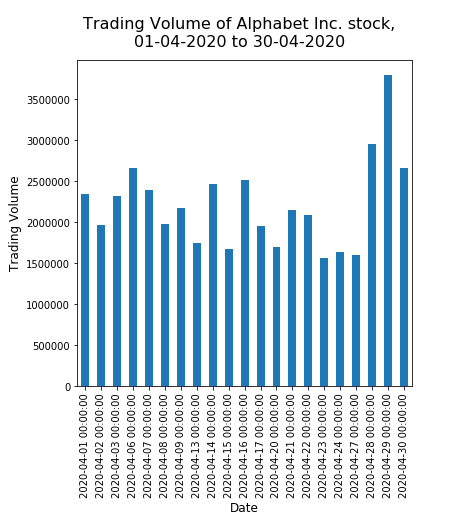﻿ Pandas: Create a bar plot of one column versus another column - w3resource# Pandas: Create a bar plot of one column versus another column

## Pandas: Plotting Exercise-3 with Solution

Write a Pandas program to create a bar plot of the trading volume of Alphabet Inc. stock between two specific dates.

In the context of a single stock trading on a stock exchange, the volume is commonly reported as the number of shares that changed hands during a given day. The transactions are measured on stocks, bonds, options contracts, futures contracts and commodities.

Use the alphabet_stock_data.csv file to extract data.

alphabet_stock_data:

alphabet_stock_data Table

Date Open High Low Close Adj Close Volume
2020-04-01 1122 1129.689941 1097.449951 1105.619995 1105.619995 2343100
2020-04-02 1098.26001 1126.859985 1096.400024 1120.839966 1120.839966 1964900
2020-04-03 1119.015015 1123.540039 1079.810059 1097.880005 1097.880005 2313400
2020-04-06 1138 1194.660034 1130.939941 1186.920044 1186.920044 2664700
... ... ... ... ... ... ...
... ... ... ... ... ... ...
2020-09-29 1470.390015 1476.662964 1458.805054 1469.329956 1469.329956 978200
2020-09-30 1466.800049 1489.75 1459.880005 1469.599976 1469.599976 1700600

Sample Solution:

Python Code :

``````import pandas as pd
import matplotlib.pyplot as plt
start_date = pd.to_datetime('2020-4-1')
end_date = pd.to_datetime('2020-4-30')
df['Date'] = pd.to_datetime(df['Date'])
new_df = (df['Date']>= start_date) & (df['Date']<= end_date)
df1 = df.loc[new_df]
df2 = df1.set_index('Date')
plt.figure(figsize=(6,6))
plt.suptitle('Trading Volume of Alphabet Inc. stock,\n01-04-2020 to 30-04-2020', fontsize=16, color='black')
plt.xlabel("Date",fontsize=12, color='black')
df2['Volume'].plot(kind='bar');
plt.show()
``````

Sample Output:

``````

Python Code Editor:

Have another way to solve this solution? Contribute your code (and comments) through Disqus.

What is the difficulty level of this exercise?

Test your Python skills with w3resource's quiz

﻿

## Python: Tips of the Day

Trenary operator:

```>>> "Python ROCK" if True else " I AM GRUMPY"
"Python ROCK"
```